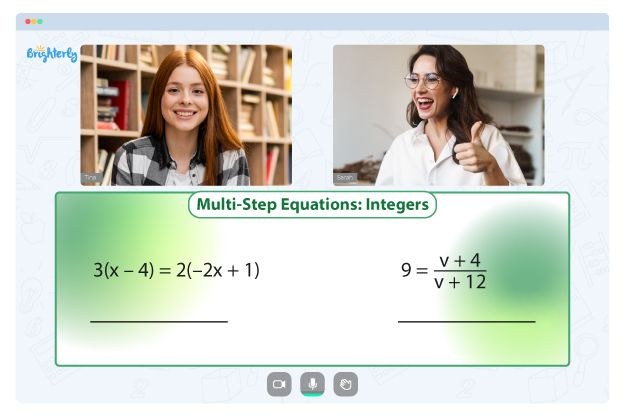# Equations With Fractions Worksheets

Equations and fractions can seem complicated for young learners. The only way to get out of this rut is by utilizing a comprehensive worksheet filled with varied exercises and problems to solve. Our solve equations with fractions worksheet is an average student’s one-stop guide to learning math. Over time, they will master basic math skills and be able to tackle equational and fractional problems.

## About Solving Equations with Fractions Worksheet

The one step equations with fractions worksheet covers everything students need to know about equations and fractions. With an equations with fractions worksheet, students learn how to make use of solving equations with fractions and decimals worksheet. More importantly, the tool teaches your kid math skills such as simplification and problem-solving. At the end of the worksheet, you can find the answers to the exercises to guide your marking.

Math for Kids

Is Your Child Struggling With Math?
1:1 Online Math Tutoring## Benefits of Solving an Equations with Fractions Worksheet

These worksheets are comprehensive and thus, the best option for any student looking to improve their math skills. The exercises are varied, ensuring that young learners get to experiment with different kinds of fractions and equations. It covers more than just one-step equations; a kid can also use a two step equations with fractions worksheet. At the end of the day, your child will learn how to work with mixed fractions, whole fractions, improper fractions, and so on. More importantly, they’ll be able to apply everything they’ve learned to real-life scenarios.### Equations With Fractions Worksheets PDF

Equations With Fractions Worksheet### Equations With Fractions Worksheets PDF

Solve Equations With Fractions Worksheet### Equations With Fractions Worksheets PDF

Solving Equations With Fractions Worksheet### Equations With Fractions Worksheets PDF

Two Step Equations With Fractions Worksheet

### More Fractions Worksheets

Need help with Equations?• Does your child need extra assistance with mastering equations?
• Start learning with an online tutor.

Is your child finding it hard to grasp equations? An online tutor could be of help.